【学习笔记】PYTHON数据分析与展示(北理工 嵩天) – Python量化投资

# 【学习笔记】PYTHON数据分析与展示(北理工 嵩天)

## 0 数据分析之前奏

Anaconda：一个集合，包括conda、某版本Python、一批第三方库等

-支持近800个第三方库

-适合科学计算领域

-包含多个主流工具

-开源免费

-跨平台

conda

-一个工具，用于包管理和环境管理

-包管理与pip类似，管理Python第三方库

-环境管理能够允许用户使用不同版本的Python，并能灵活切换

conda将工具、第三方库、Python版本、conda都当作包，同等对待

conda有命令行工具

C:\Users\king\Anaconda3\Scripts\conda.exe –version 可以查看conda版本

conda update conda    升级conda

-是一个功能强大的交互式shell

-适合进行交互式数据可视化和GUI相关应用

IPython几个技巧

？：可以在变量或者函数前面加？获得通用信息

%run ：可以执行.py程序 注意：%run在一个空的命名空间执行%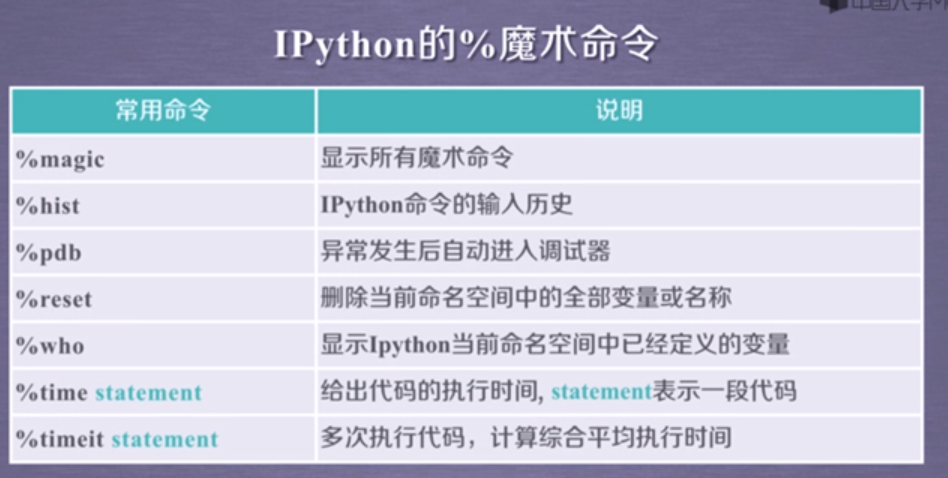trouble shooting:

## 1. 数据分析之表示

### 1.1 NumPy库入门

NumPy的数组对象：ndarray

NumPy是一个开源的Python科学计算基础库

-一个强大的N维数组对象ndarray

-广播函数功能

-整合c/c++/fortran代码的工具

-线性代数、傅里叶变换、随机数生成等功能

NumPy是SciPy、Pandas等数据处理或科学计算库的基础

ndarray是一个多维数组，由两部分组成，要求数组元素类型相同，数组下标从0开始

-元数据（数据维度，数据类型等）

-实际数据

np.array()                  –ndarray别名是array

-科学计算涉及大量数据，对性能和存储都有较高要求

-对元素类型精细定义，有助于numpy合理使用存储空间并优化性能，有助于程序员对程序规模由合理评估

ndarray数组也可以由非同质对象构成

ndarray数组的创建和变换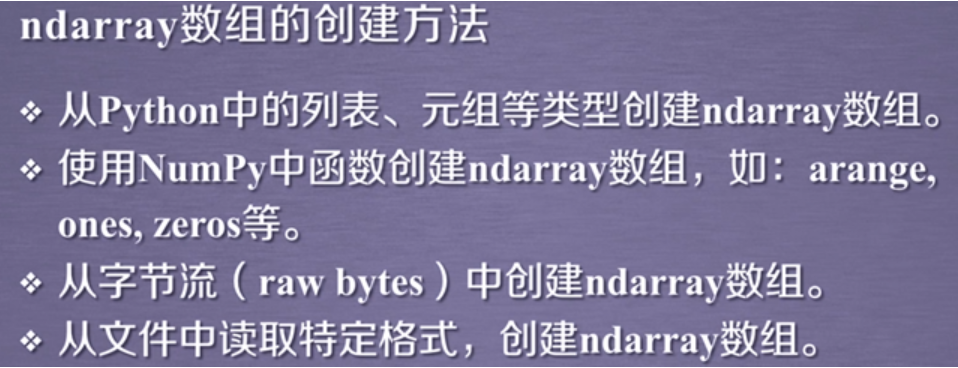1) 从python列表元组等类型创建数组

x = np.array(list/tuple)

x = np.array(list/tuple,dtype=np.float32)   不指定类型的时候numpy自动关联一个合适的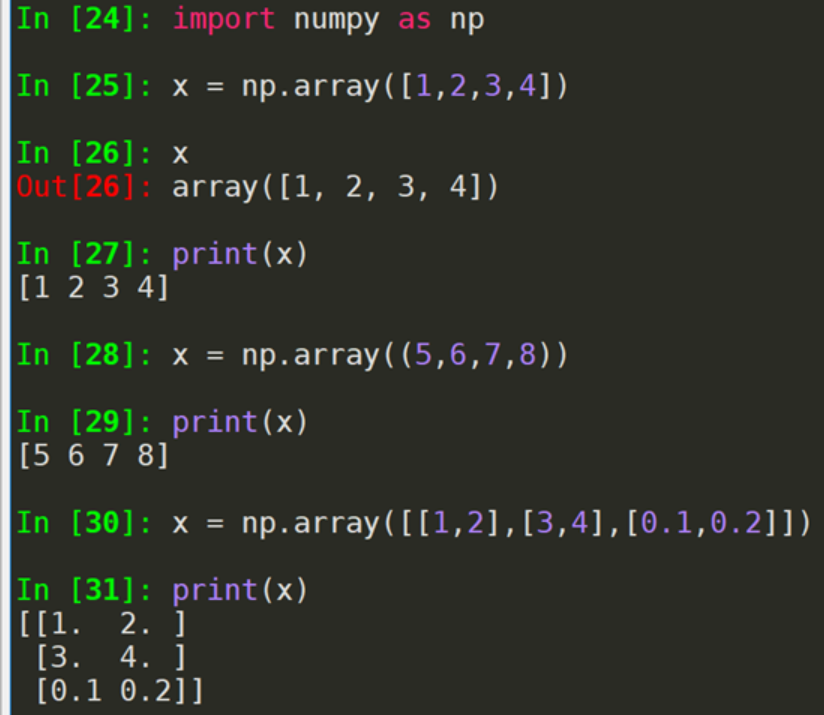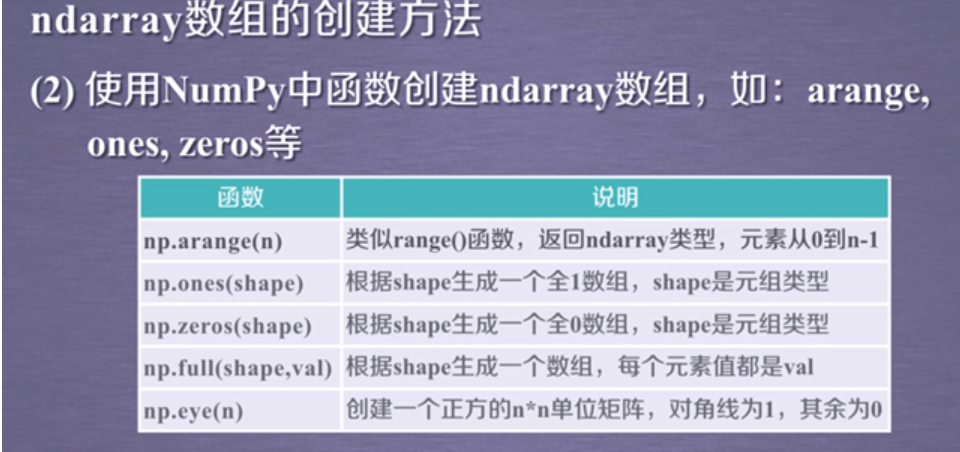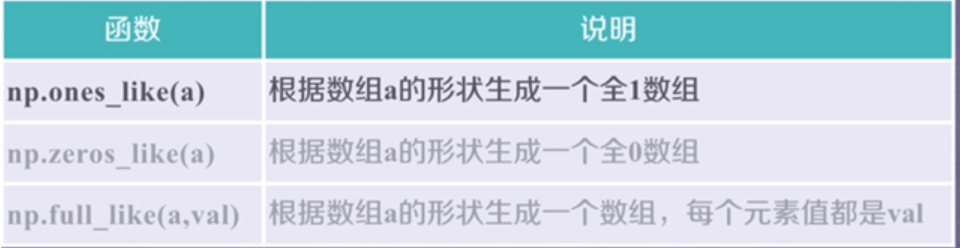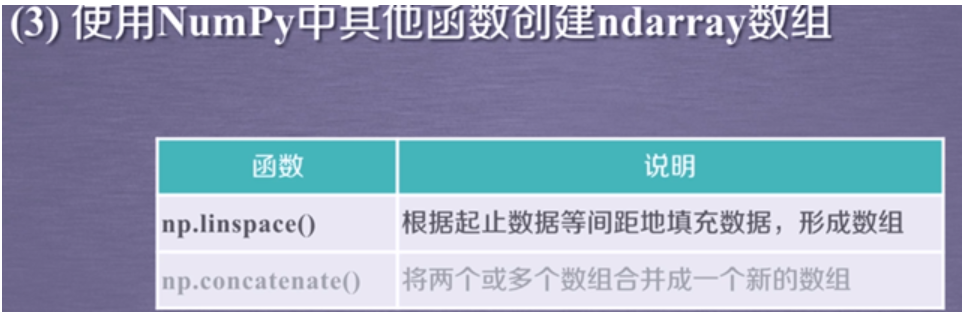除了arange函数，其他都是浮点数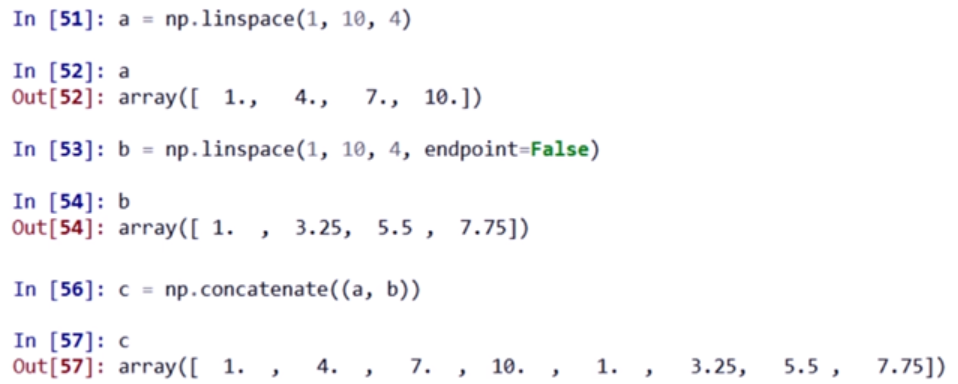ndarray数组的变换：维度变换、元素类型变换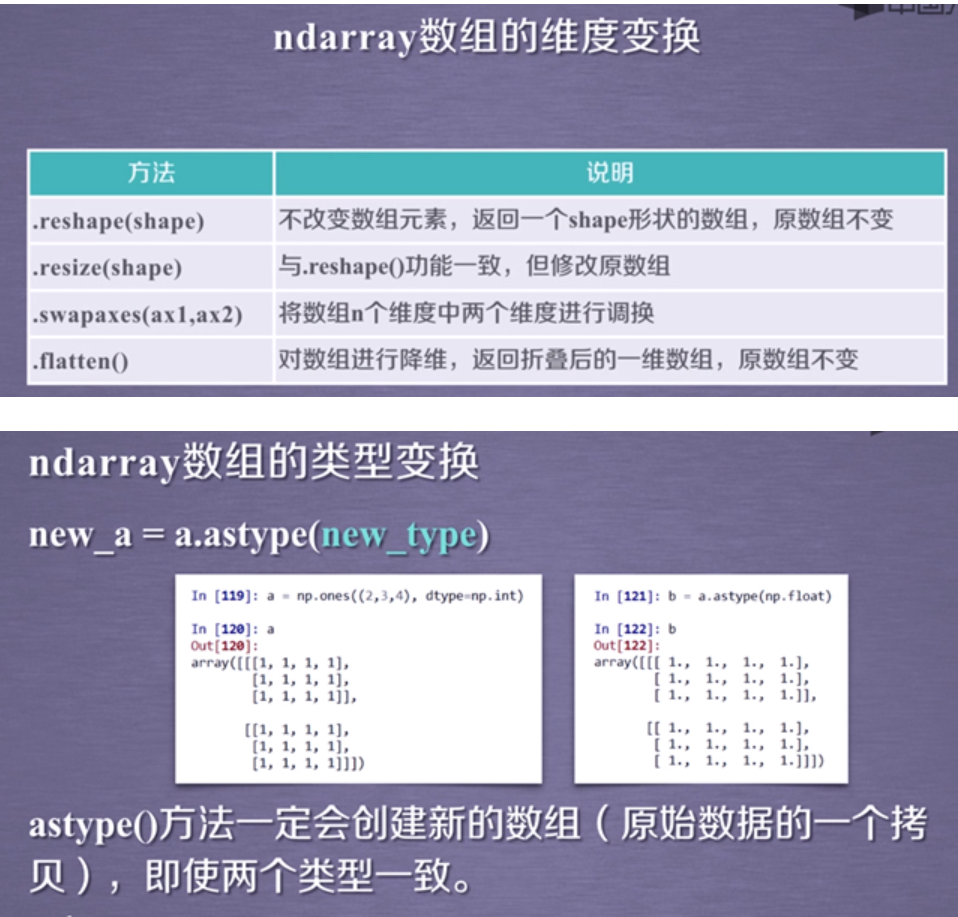ndarray数组向列表变换

ls = a.tolist()

ndarray数组的操作

ndarray一维数组的索引和列表一样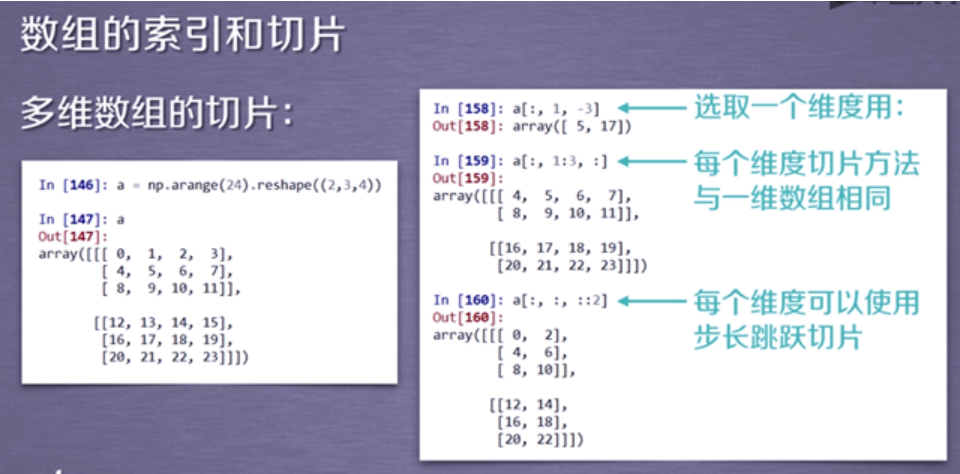ndarray数组的运算

maximum等经过运算存在数据类型隐式转换

### 1.2 NumPy数据存取与函数

csv只能存储读取一维和二维数据，这是它的局限

-frame 文件、字符串  sep：数据分隔符，如果是空串，写入文件为二进制 format：写入数据的格式-dtype:读取的数据类型  count：读入元素的个数-1表示全部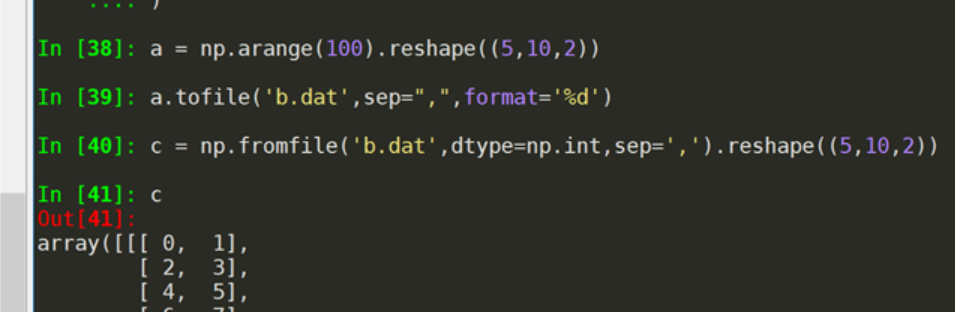NumPy的便捷文件存取

np.save(fname,array)或者np.savez(fname,array)

-fname:文件名以.npy或者.npz

NumPy的随机数函数

NumPy的random子库 np.random.*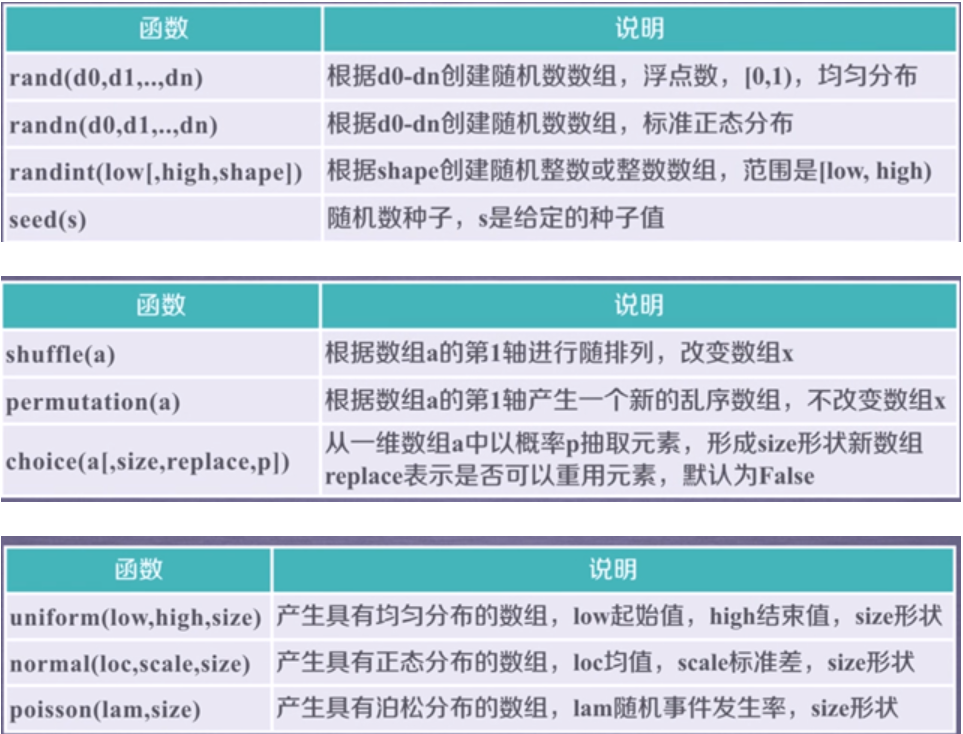NumPy的统计函数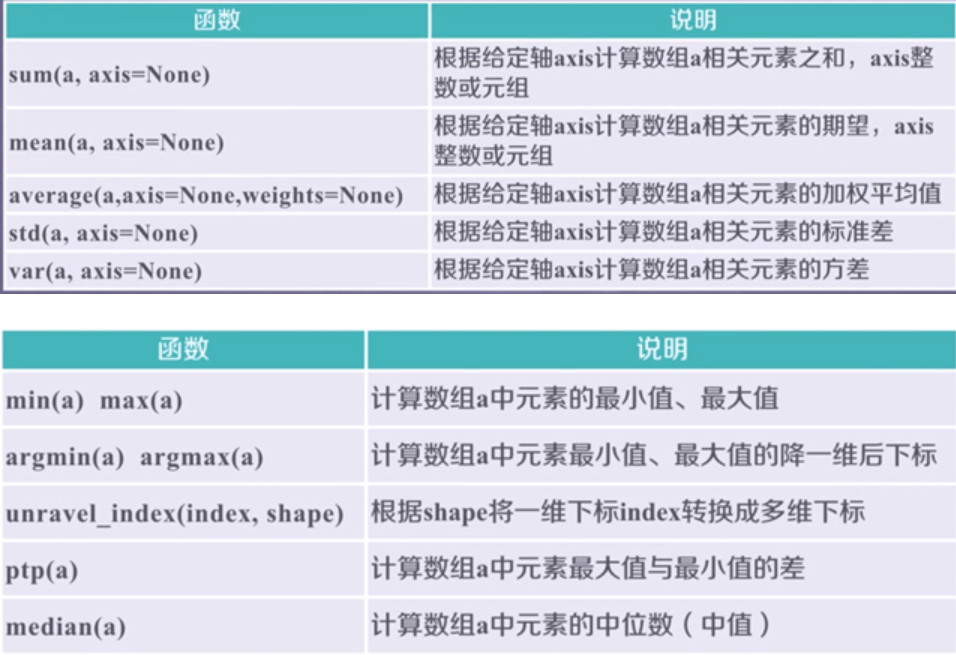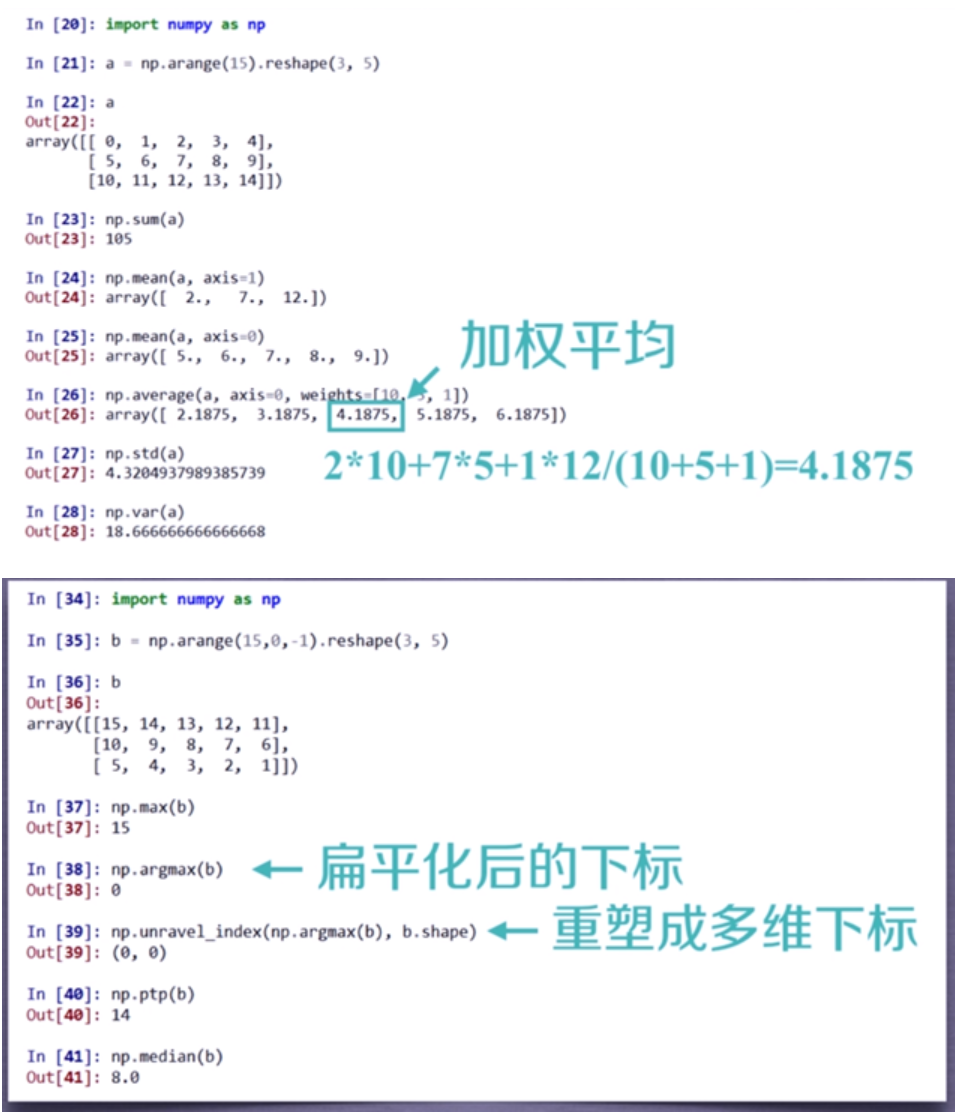NumPy的梯度函数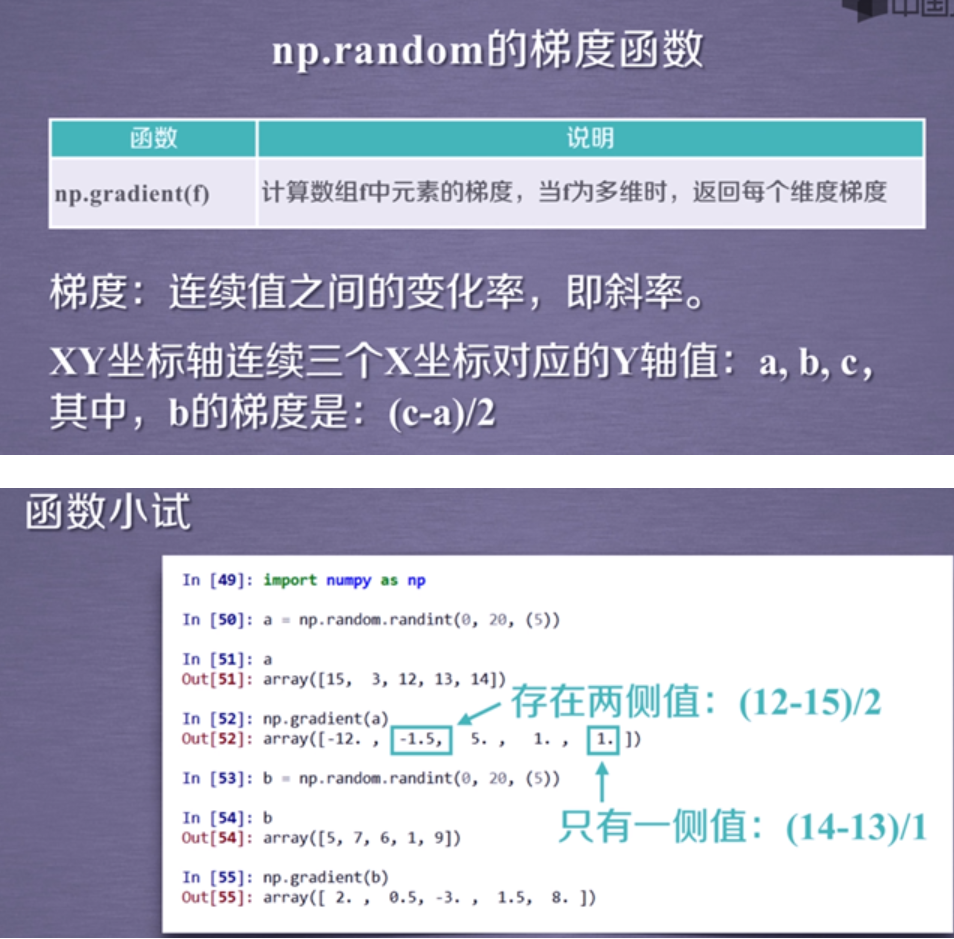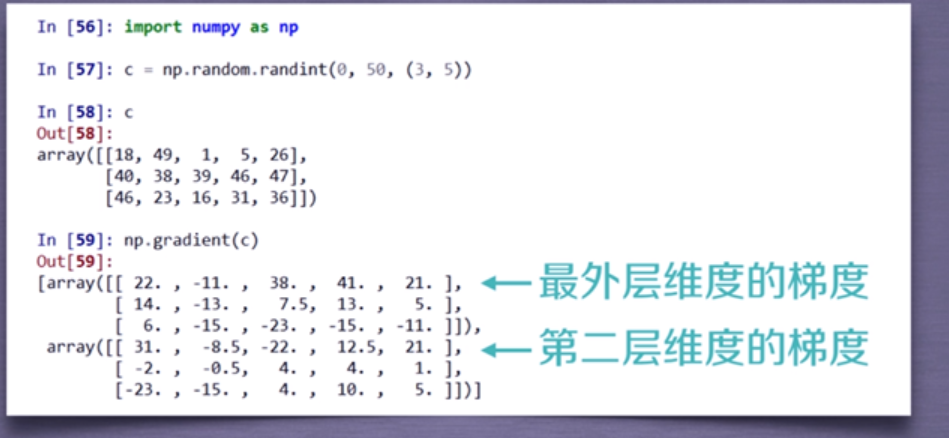### 1.3 实例1：图像的手绘效果

RGB色彩表示

PIL库  处理图像的第三方库 pip install pillow                   from PIL import Image

Image是PIL库中代表图像的类(对象)

from PIL import Image
import numpy as np

a = np.asarray(Image.open('./beijing.jpg').convert('L')).astype('float')

depth = 10.                      # (0-100)
A = np.sqrt(grad_x**2 + grad_y**2 + 1.)
uni_z = 1./A

vec_el = np.pi/2.2                   # 光源的俯视角度，弧度值
vec_az = np.pi/4.                    # 光源的方位角度，弧度值
dx = np.cos(vec_el)*np.cos(vec_az)   #光源对x 轴的影响
dy = np.cos(vec_el)*np.sin(vec_az)   #光源对y 轴的影响
dz = np.sin(vec_el)              #光源对z 轴的影响

b = 255*(dx*uni_x + dy*uni_y + dz*uni_z)     #光源归一化
b = b.clip(0,255)

im = Image.fromarray(b.astype('uint8'))  #重构图像
im.save('./beijingHD.jpg')## 2. 数据分析与展示

### 2.1 Matplotlib库入门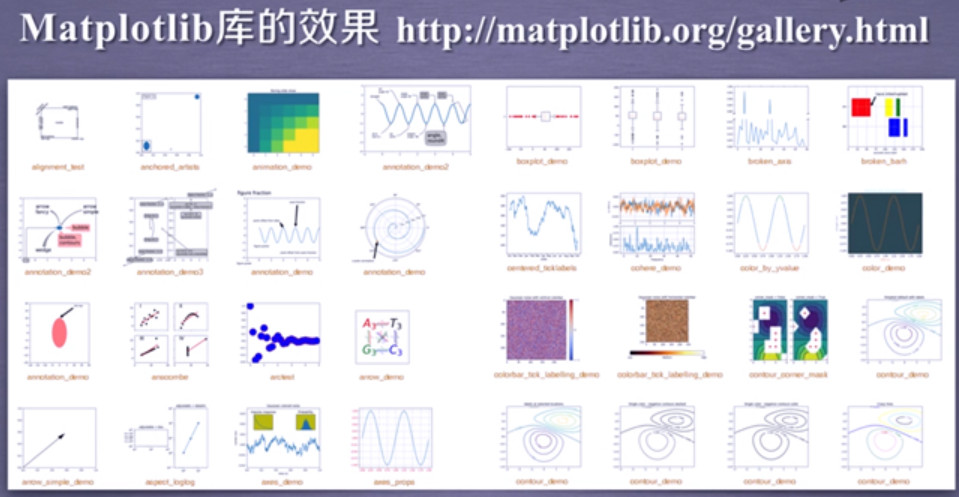matplotlib.pyplot是绘制各类可视化图形的命令子库，相当于快捷方式

import matplotlib.pyplot as plt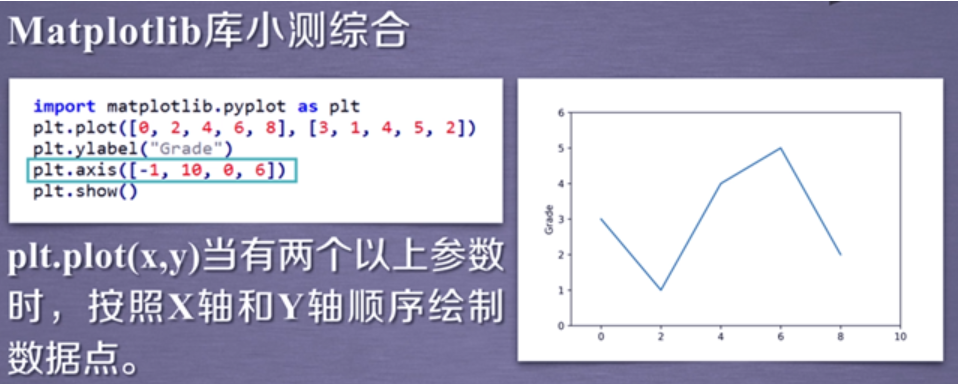plt.savefig(‘test’,dpi=600) #保存为png文件

pylot的plot函数

plt.plot(x,y,format_string,**kwargs)

-format_string 表示曲线的格式字符串   **kwargs表示第二组或更多(x,y,format_string)   当绘制多条曲线x不能省略，当绘制一条时候可以省略x用索引值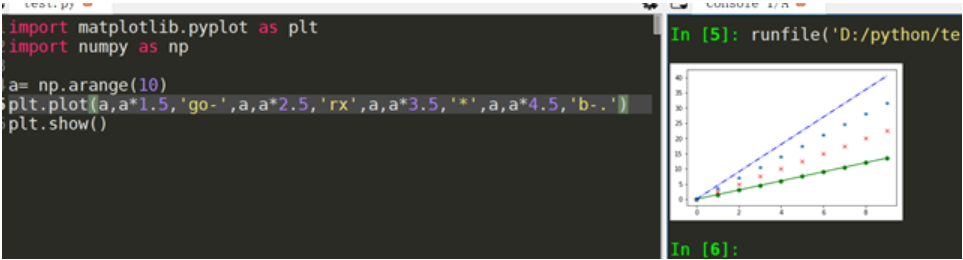format_string由颜色字符、风格字符、标记字符组成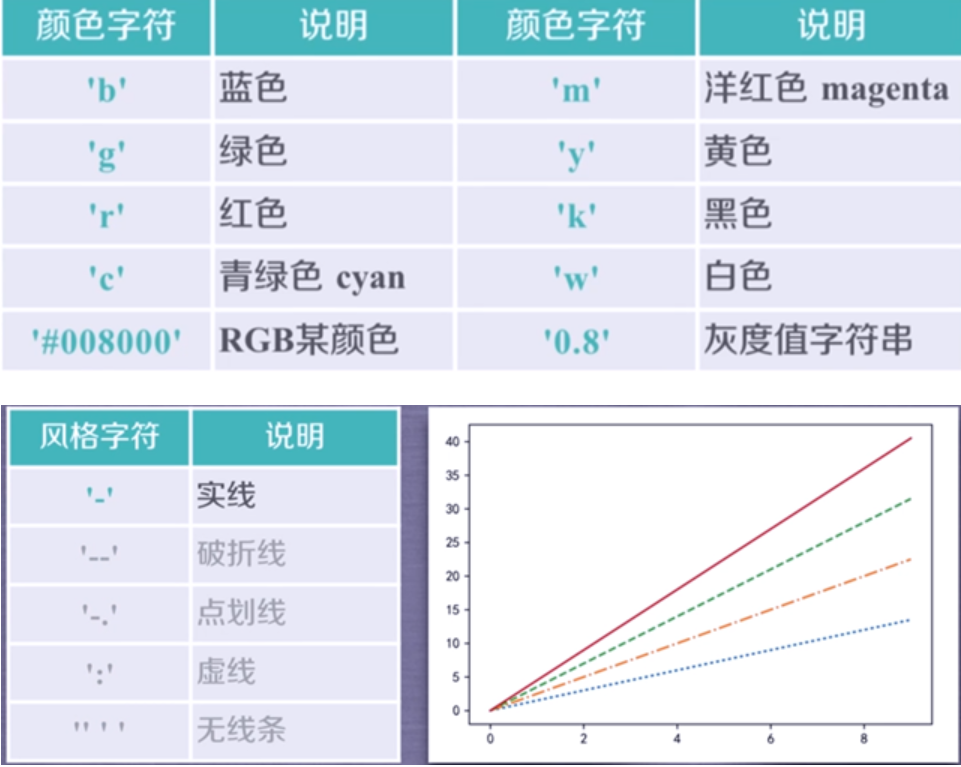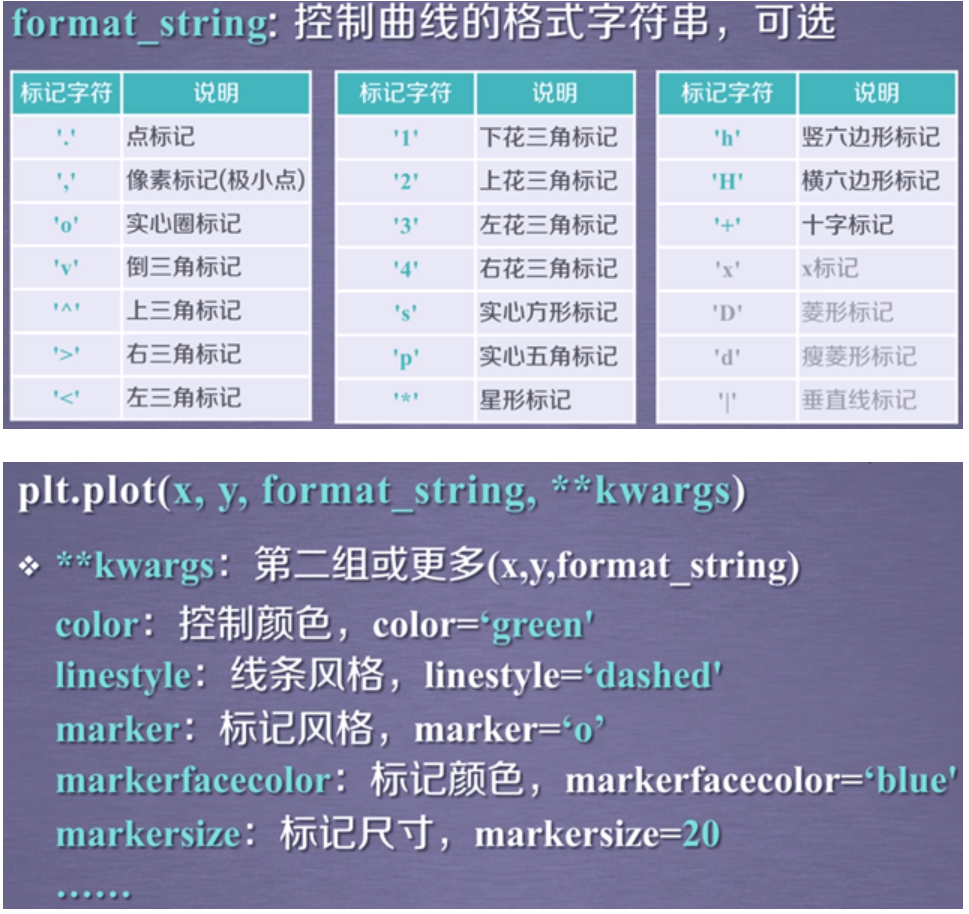pyplot的中文显示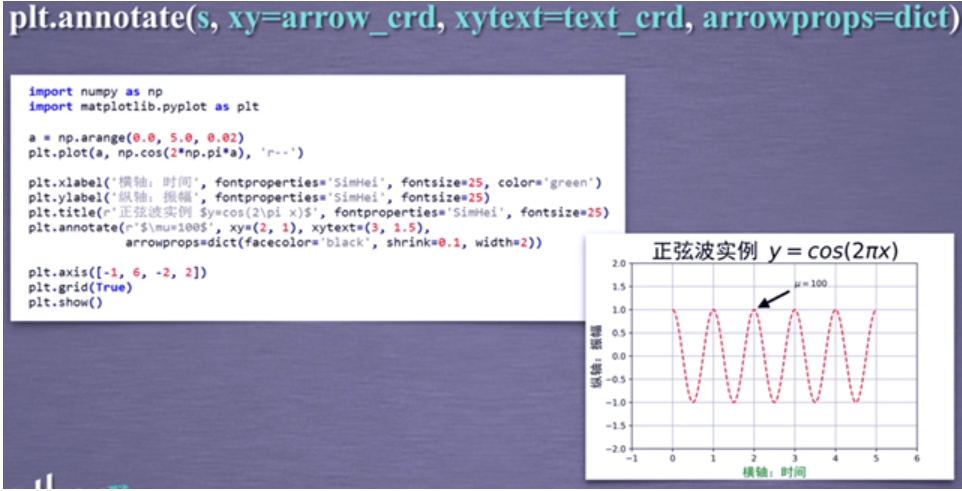pylot子绘图区域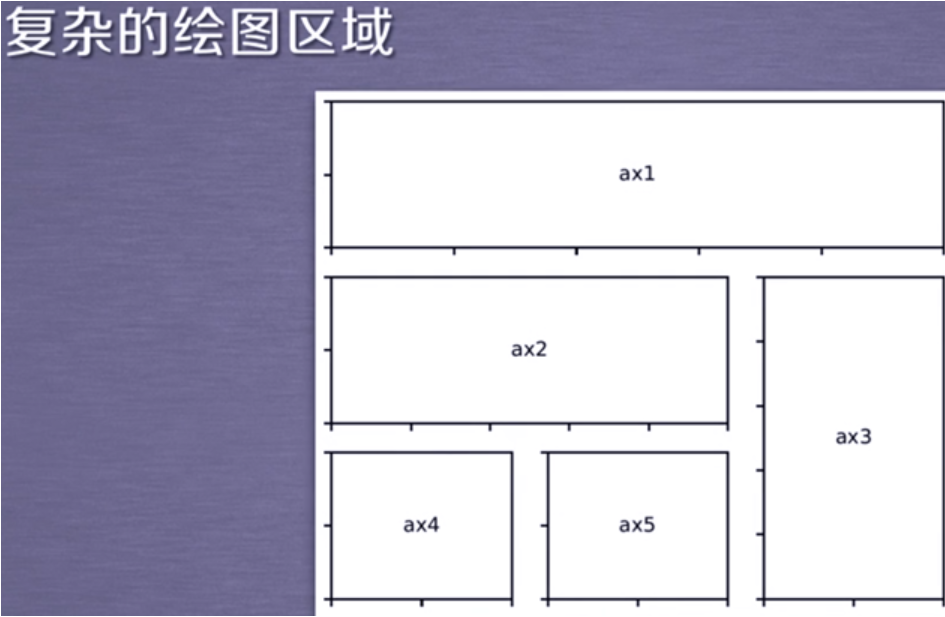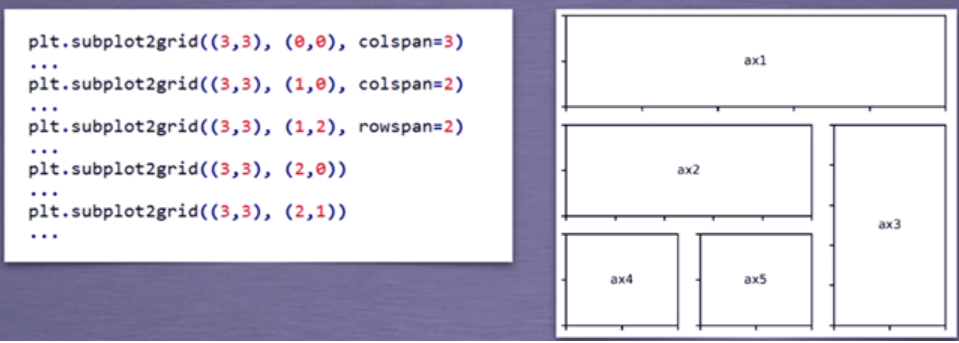这种方法每次都要写（3，3），用另外一种方法GridSpec类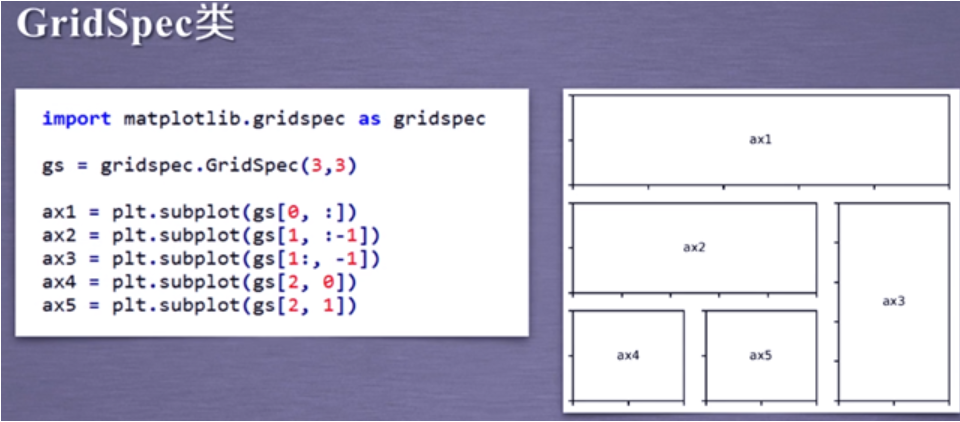pylot饼图绘制

pylot直方图绘制

pylot极坐标绘制

pyplot散点图绘制

### 2.3 实例2：引力波的绘制

import numpy as np
import matplotlib.pyplot as plt
from scipy.io import wavfile

#reftime, ref_H1 = np.genfromtxt('GW150914_4_NR_waveform_template.txt').transpose()
reftime, ref_H1 = np.genfromtxt('wf_template.txt').transpose() #使用python123.io下载文件

htime_interval = 1/rate_h
ltime_interval = 1/rate_l
fig = plt.figure(figsize=(12, 6))

# 丢失信号起始点
htime_len = hstrain.shape/rate_h
htime = np.arange(-htime_len/2, htime_len/2 , htime_interval)
plth.plot(htime, hstrain, 'y')
plth.set_xlabel('Time (seconds)')
plth.set_ylabel('H1 Strain')
plth.set_title('H1 Strain')

ltime_len = lstrain.shape/rate_l
ltime = np.arange(-ltime_len/2, ltime_len/2 , ltime_interval)
pltl.plot(ltime, lstrain, 'g')
pltl.set_xlabel('Time (seconds)')
pltl.set_ylabel('L1 Strain')
pltl.set_title('L1 Strain')

pltref.plot(reftime, ref_H1)
pltref.set_xlabel('Time (seconds)')
pltref.set_ylabel('Template Strain')
pltref.set_title('Template')
fig.tight_layout()

plt.savefig("Gravitational_Waves_Original.png")
plt.show()
plt.close(fig)


## 3. 数据分析之概要

### 3.1 Pandas库入门

Pandas提供高性能易用数据类型和分析工具

import pandas as pd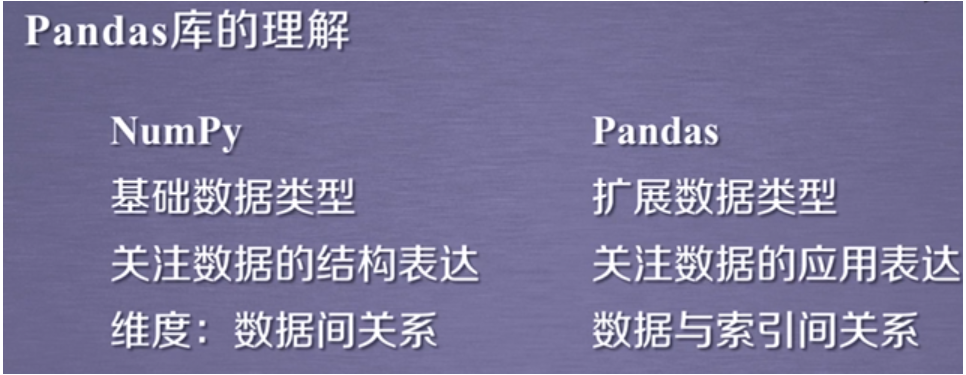Pandas库的Series类型     –一维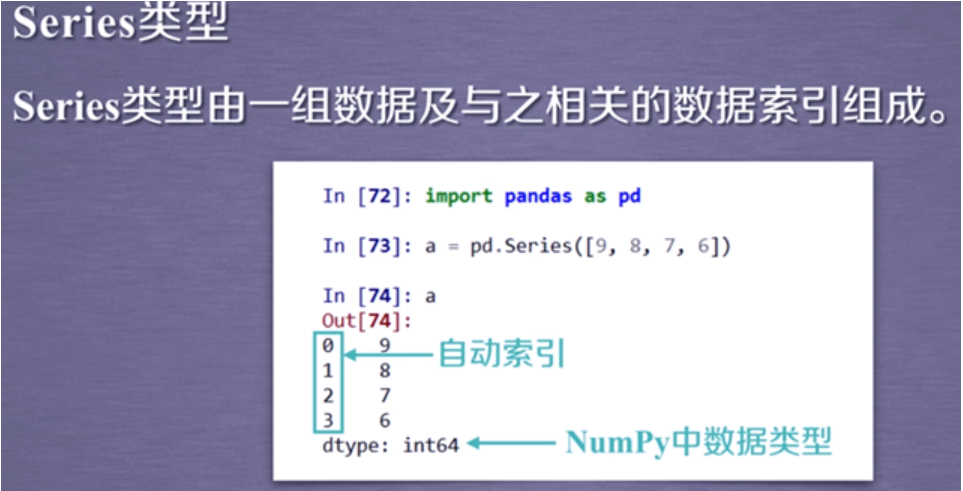也支持自定义索引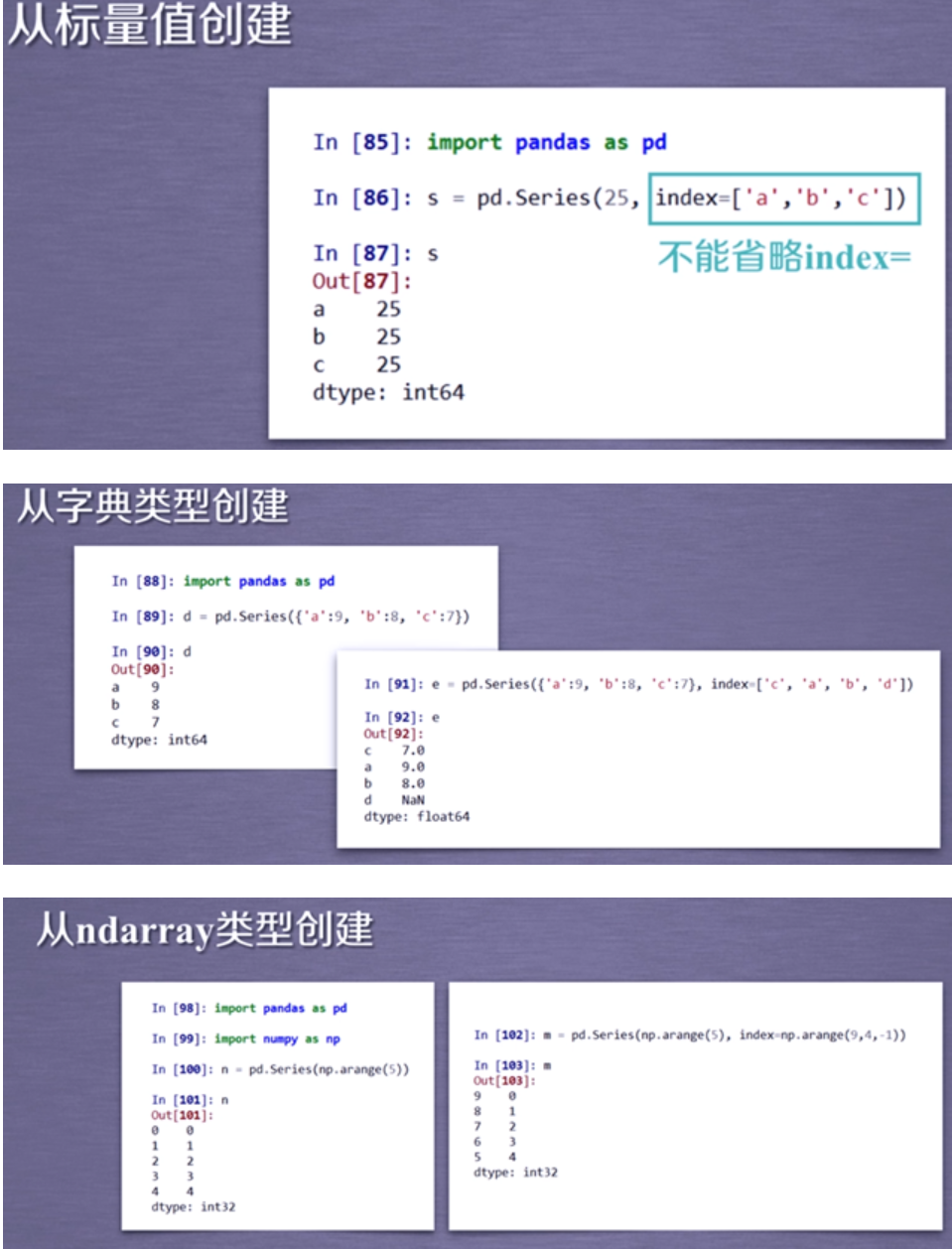b.index  b.values   获得索引和数据  类似ndarray和字典

Pandas库的DataFrame类型  –二维

DataFrame基本操作类似Series，根据行列索引

Pandas库的数据数据类型操作

Series只有0轴    DataFrame由0轴 1轴，drop默认操作0轴

Pandas库的数据类型运算

### 3.2 Pandas数据特征分析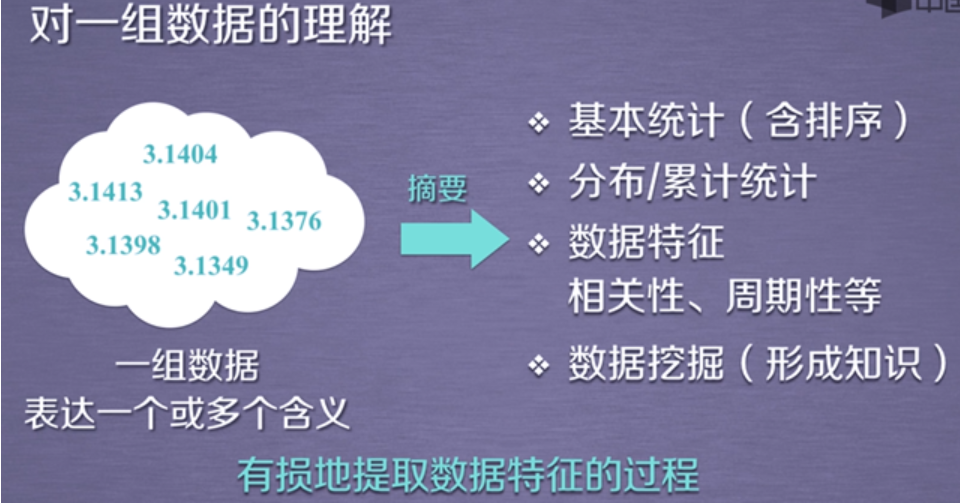https://www.cnblogs.com/yongestcat/p/11464694.html

「点点赞赏，手留余香」

还没有人赞赏，快来当第一个赞赏的人吧！
Python
Python
0 条回复 A 作者 M 管理员
所有的伟大，都源于一个勇敢的开始！